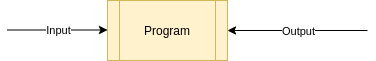# Part-1: What are Algorithms?

## What is an Algorithm?

In programming term an Algorithm is consists of set of rules or instructions to solve a problem. Some of the examples of Algorithms are:

• Writing a program to make a coffee
• Writing a program to perform sum of two numbers
• Writing a program that takes some input and produce some output etc...

In easy term, Algorithm takes some inputs and produce some output.A program (or Algorithm) is consists of number of statements. Each statement is consists of number of operations.

Let's look at the following example to understand:

``````# function takes two arguments (input) a and b
function sum(a, b) {

// this is a statement
// below statement has one operation (addition)
return a + b;
}

echo sum(1, 2); // output: 3​``````

Example: A google search engine is a type of Algorithm that takes some user input as a search query and searches its database to find matched results and then outputs the results on browser screen.

## What are some of the characteristics of an Algorithm?

Not all programs are considered as an Algorithms. An Algorithm should have some of the following characteristics:

• Algorithm should be clear and unambiguos.
• An Algorithm should have one or more well-defined inputs.
• An Algorithm should have one or more well-defined outputs and should match desired output.
• Algorithms must terminate after a finite number of steps or instructions.

## How to write an Algorithm?

There are no good ways to write Algorithms. They depends on type of problem and resource available. You can learn basic programming concepts like:

• language syntax
• declaring and storing different types of variables
• using basic code constructs like
• loops (do, while, for)
• flow-control (if-else or switch)

You can write Algorithm in any programming language you just need to learn basic code constructs as shown above to get started with. Let's take simple example.

Design an Algorithm to add two numbers and display the results. First of all we will write all the instructions we need in order to complete the algorithm.

• START
• Define two variables a and b
• Define third variable that stores sum of a and b
• Print the result of variable c
• END

Now, let say for learning purpose I am choosing javascript language to write above algorithm. Here is the actual program that solves above problem:

``````// define two variables a and b
const a = 1, b = 2;

// define third variable that stores sum of a and b
const c = a + b;

// display output of variable c
console.log(c); // prints output: 3​``````

That is it we have wrote our first algorithm. A problem can be solved with multiple solutions because an Algorithm depends on resources we need to pick the best solution that we find for a given problem.

## Why we need of Big O notation?

Any programming problem or algorithm depends on two major things:

• Time: Amount of time it takes to run the specific program
• Space: Amount of space it takes to run the specific program

If you write a program that takes lots of memory or space in your computer it will crash. Similarly, if you write a program that takes forever to output the results it is considered as a bad program.

Big O notation help us find upper, middle and lower values for time and space and therefore you can make decisions on which solution is bettter for a given programming problem.

In next tutorial we will learn about Big O Notation and how does it help us writing a better computer programs.